# Supporting Competing APIs in Scala – Can Better Package Factoring Help?

| Feedback

On and off over the last year, I’ve been working on a library of tree and map classes in Scala that happen to make use of some algebraic structures (mostly monoids or related concepts). In my initial implementations, I made use of the popular algebird variations on monoid and friends. In their incarnation as an algebird PR this was uncontroversial to say the least, but lately I have been re-thinking them as a third-party Scala package.

This immediately raised some interesting and thorny questions: in an ecosystem that contains not just algebird, but other popular alternatives such as cats and scalaz, what algebra API should I use in my code? How best to allow the library user to interoperate with the algebra libray of their choice? Can I accomplish these things while also avoiding any problematic package dependencies in my library code?

In Scala, the second question is relatively straightforward to answer. I can write my interface using implicit conversions, and provide sub-packages that provide such conversions from popular algebra libraries into the library I actually use in my code. A library user can import the predefined implicit conversions of their choice, or if necessary provide their own.

So far so good, but that leads immediately back to the first question – what API should I choose to use internally in my own library?

One obvious approach is to just pick one of the popular options (I might favor cats, for example) and write my library code using that. If a library user also prefers cats, great. Otherwise, they can import the appropritate implicit conversions from their favorite alternative into cats and be on their way.

But this solution is not without drawbacks. Anybody using my library will now be including cats as a transitive dependency in their project, even if they are already using some other alternative. Although cats is not an enormous library, that represents a fair amount of code sucked into my users’ projects, most of which isn’t going to be used at all. More insidiously, I have now introduced the possiblity that the cats version I package with is out of sync with the version my library users are building against. Version misalignment in transitive dependencies is a land-mine in project builds and very difficult to resolve.

A second approach I might use is to define some abstract algebraic traits of my own. I can write my libraries in terms of this new API, and then provide implicit conversions from popular APIs into mine.

This approach has some real advantages over the previous. Being entirely abstract, my internal API will be lightweight. I have the option of including only the algebraic concepts I need. It does not introduce any possibly problematic 3rd-party dependencies that might cause code bloat or versioning problems for my library users.

Although this is an effective solution, I find it dissatisfying for a couple reasons. Firstly, my new internal API effectively represents yet another competing algebra API, and so I am essentially contributing to the proliferating-standards antipattern.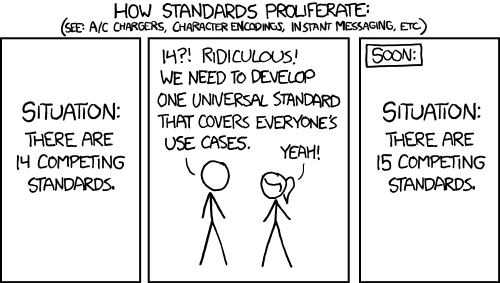Secondly, it means that I am not taking advantage of community knowledge. The cats library embodies a great deal of cumulative human expertise in both category theory and Scala library design. What does a good algebra library API look like? Well, it’s likely to look a lot like cats of course! The odds that I end up doing an inferior job designing my little internal vanity API are rather higher than the odds that I do as well or better. The best I can hope for is to re-invent the wheel, with a real possibility that my wheel has corners.

Is there a way to resolve this unpalatable situation? Can we design our projects to both remain flexible about interfacing with multiple 3rd-party alternatives, but avoid effectively writing yet another alternative for our own internal use?

I hardly have any authoritative answers to this problem, but I have one idea that might move toward a solution. As I alluded to above, when I write my libraries, I am most frequently only interested in the API – the abstract interface. If I did go with writing my own algebra API, I would seek to define purely abstract traits. Since my intention is that my library users would supply their own favorite library alternative, I would have no need or desire to instantiate any of my APIs. That function would be provided by the separate sub-projects that provide implicit conversions from community alternatives into my API.

On the other hand, what if cats and algebird factored their libraries in a similar way? What if I could include a sub-package like cats-kernel-api, or algebird-core-api, which contained only pure abstract traits for monoid, semigroup, etc? Then I could choose my favorite community API, and code against it, with much less code bloat, and a much reduced vulnerability to any versioning drift. I would still be free to provide implicit conversions and allow my users to make their own choice of library in their projects.

Although I find this idea attractive, it is certainly not foolproof. For example, there is never a way to guarantee that versioning drift won’t break an API. APIs such as cats and algebird are likely to be unusually amenable to this kind of approach. After all, their interfaces are primarily driven by underlying mathematical definitions, which are generally as stable as such things ever get. However, APIs in general tend to be significantly more stable than underlying code. And the most-stable subsets of APIs might be encoded as traits and exposed this way, allowing other more experimental API components to change at a higher frequency. Perhaps library packages could even be factored in some way such as library-stable-api and library-unstable-api. That would clearly add a bit of complication to library trait hierarchies, but the payoff in terms of increased 3rd-party usability might be worth it.

# Using Minimum Description Length to Optimize the ‘K’ in K-Medoids

| Feedback

Applying many popular clustering models, for example K-Means, K-Medoids and Gaussian Mixtures, requires an up-front choice of the number of clusters – the ‘K’ in K-Means, as it were. Anybody who has ever applied these models is familiar with the inconvenient task of guessing what an appropriate value for K might actually be. As the size and dimensionality of data grows, estimating a good value for K rapidly becomes an exercise in wild guessing and multiple iterations through the free-parameter space of possible K values.

There are some varied approaches in the community for addressing the task of identifying a good number of clusters in a data set. In this post I want to focus on an approach that I think deserves more attention than it gets: Minimum Description Length.

Many years ago I ran across a superb paper by Stephen J. Roberts on anomaly detection that described a method for automatically choosing a good value for the number of clusters based on the principle of Minimum Description Length. Minimum Description Length (MDL) is an elegant framework for evaluating the parsimony of a model. The Description Length of a model is defined as the amount of information needed to encode that model, plus the encoding-length of some data, given that model. Therefore, in an MDL framework, a good model is one that allows an efficient (i.e. short) encoding of the data, but whose own description is also efficient (This suggests connections between MDL and the idea of learning as a form of data compression).

For example, a model that directly memorizes all the data may allow for a very short description of the data, but the model itself will cleary require at least the size of the raw data to encode, and so direct memorization models generaly stack up poorly with respect to MDL. On the other hand, consider a model of some Gaussian data. We can describe these data in a length proportional to their log-likelihood under the Gaussian density. Furthermore, the description length of the Gaussian model itself is very short; just the encoding of its mean and standard deviation. And so in this case a Gaussian distribution represents an efficient model with respect to MDL.

In summary, an MDL framework allows us to mathematically capture the idea that we only wish to consider increasing the complexity of our models if that buys us a corresponding increase in descriptive power on our data.

In the case of Roberts’ paper, the clustering model in question is a Gaussian Mixture Model (GMM), and the description length expression to be optimized can be written as: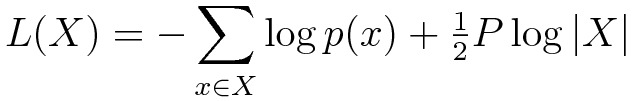In this expression, X represents the vector of data elements. The first term is the (negative) log-likelihood of the data, with respect to a candidate GMM having some number (K) of Gaussians; p(x) is the GMM density at point (x). This term represents the cost of encoding the data, given that GMM. The second term is the cost of encoding the GMM itself. The value P is the number of free parameters needed to describe that GMM. Assuming a dimensionality D for the data, then P = K(D + D(D+1)/2): D values for each mean vector, and D(D+1)/2 values for each covariance matrix.

I wanted to apply this same MDL principle to identifying a good value for K, in the case of a K-Medoids model. How best to adapt MDL to K-Medoids poses some problems. In the case of K-Medoids, the only structure given to the data is a distance metric. There is no vector algebra defined on data elements, much less any ability to model the points as a Gaussian Mixture.

However, any candidate clustering of my data does give me a corresponding distribution of distances from each data element to it’s closest medoid. I can evaluate an MDL measure on these distance values. If adding more clusters (i.e. increasing K) does not sufficiently tighten this distribution, then its description length will start to increase at larger values of K, thus indicating that more clusters are not improving our model of the data. Expressing this idea as an MDL formulation produces the following description length formula: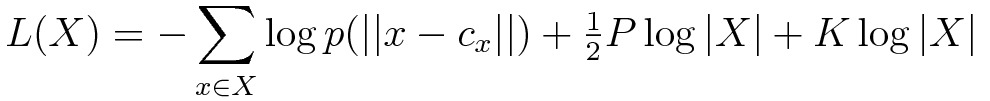Note that the first two terms are similar to the equation above; however, the underlying distribution p(||x-cx||) is now a distribution over the distances of each data element (x) to its closest medoid cx, and P is the corresponding number of free parameters for this distribution (more on this below). There is now an additional third term, representing the cost of encoding the K medoids. Each medoid is a data element, and specifying each data element requires log|X| bits (or nats, since I generally use natural logarithms), yielding an additional (K)log|X| in description length cost.

And so, an MDL-based algorithm for automatically identifying a good number of clusters (K) in a K-Medoids model is to run a K-Medoids clustering on my data, for some set of potential K values, and evaluate the MDL measure above for each, and choose the model whose description length L(X) is the smallest!

As I mentioned above, there is also an implied task of choosing a form (or a set of forms) for the distance distribution p(||x-cx||). At the time of this writing, I am fitting a gamma distribution to the distance data, and using this gamma distribution to compute log-likelihood values. A gamma distribution has two free parameters – a shape parameter and a location parameter – and so currently the value of P is always 2 in my implementations. I elaborated on some back-story about how I arrived at the decision to use a gamma distribution here and here. An additional reason for my choice is that the gamma distribution does have a fairly good shape coverage, including two-tailed, single-tailed, and/or exponential-like shapes.

Another observation (based on my blog posts mentioned above) is that my use of the gamma distribution implies a bias toward cluster distributions that behave (more or less) like Gaussian clusters, and so in this respect its current behavior is probably somewhat analogous to the G-Means algorithm, which identifies clusterings that yield Gaussian disributions in each cluster. Adding other candidates for distance distributions is a useful subject for future work, since there is no compelling reason to either favor or assume Gaussian-like cluster distributions over all kinds of metric spaces. That said, I am seeing reasonable results even on data with clusters that I suspect are not well modeled as Gaussian distributions. Perhaps the shape-coverage of the gamma distribution is helping to add some robustness.

To demonstrate the MDL-enhanced K-Medoids in action, I will illustrate its performance on some data sets that are amenable to graphic representation. The code I used to generate these results is here.

Consider this synthetic data set of points in 2D space. You can see that I’ve generated the data to have two latent clusters: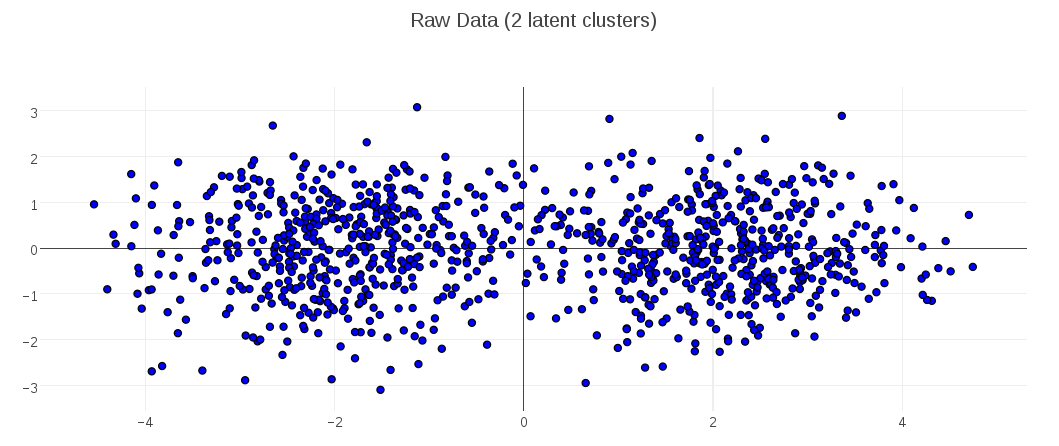I collected the description-length values for candidate K-Medoids models having 1 up to 10 clusters, and plotted them. This plot shows that the clustering with minimal description length had 2 clusters: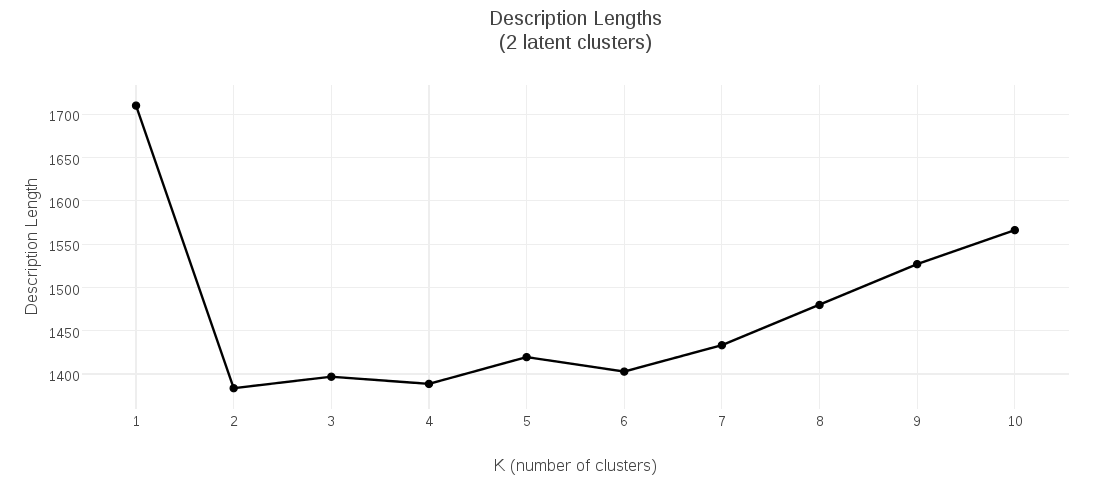When I plot that optimal clustering at K=2 (with cluster medoids marked in black-and-yellow), the clustering looks good: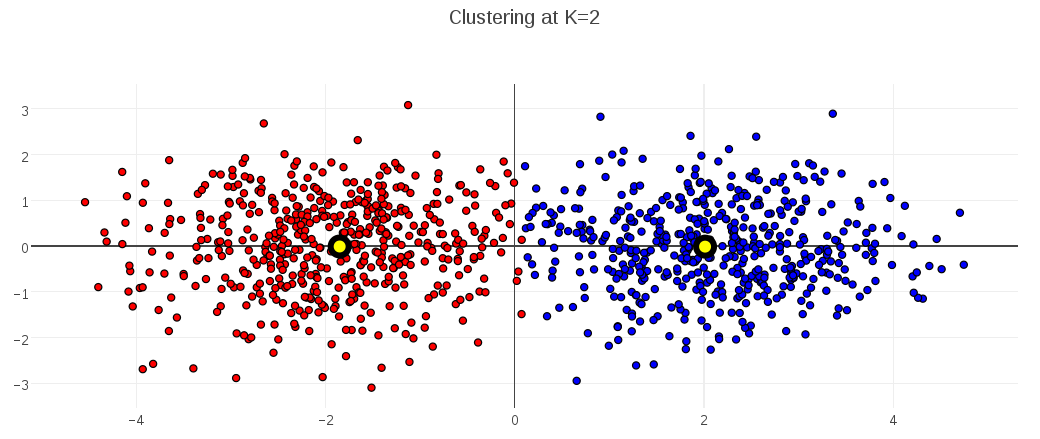To show the behavior for a different optimal value, the following plots demonstrate the MDL K-Medoids results on data where the number of latent clusters is 4: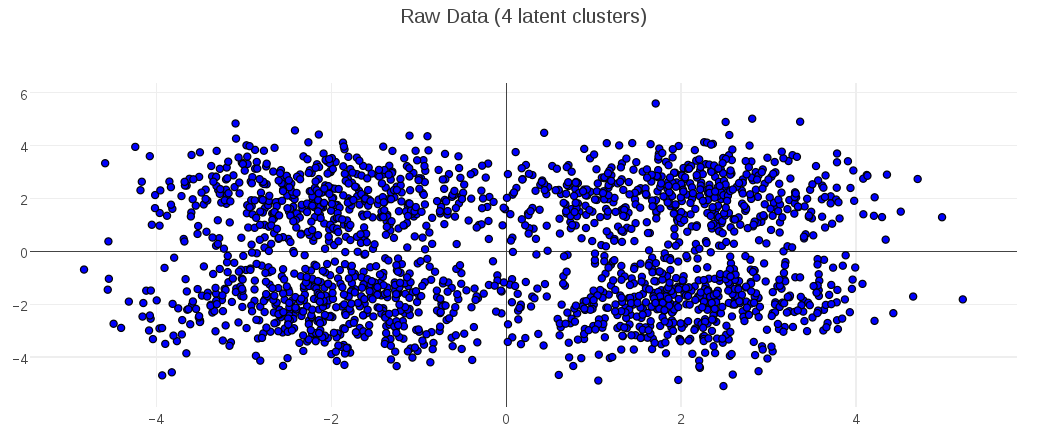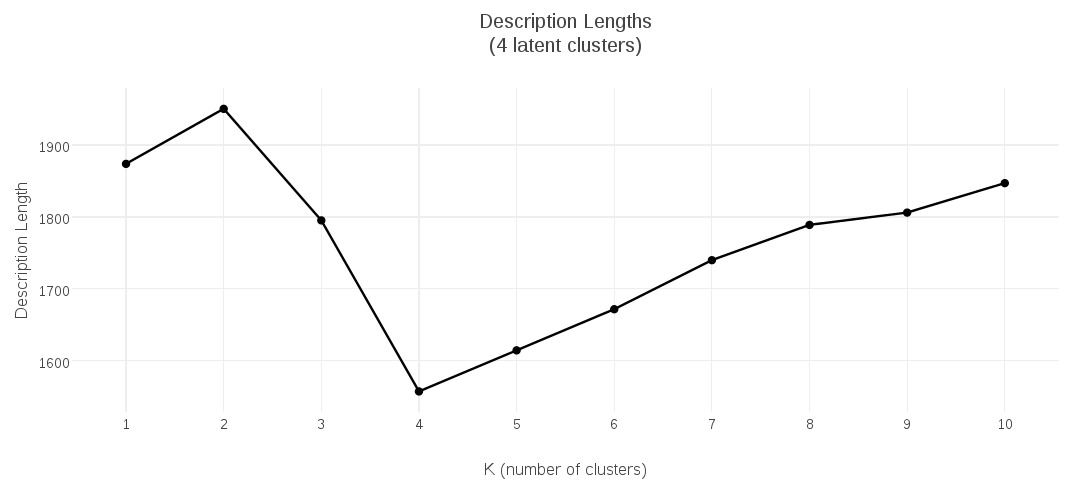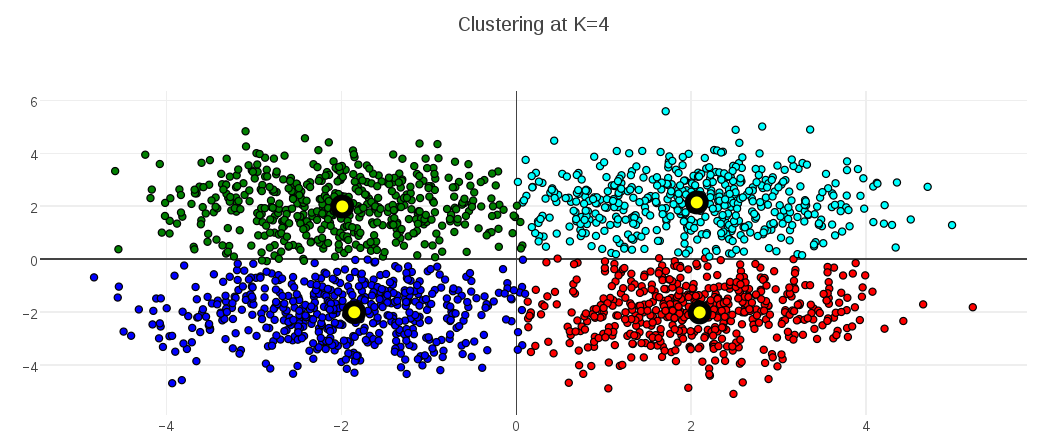A final comment on Minimum Description Length approaches to clustering – although I focused on K-Medoids models in this post, the basic approach (and I suspect even the same description length formulation) would apply equally well to K-Means, and possibly other clustering models. Any clustering model that involves a distance function from elements to some kind of cluster center should be a good candidate. I intend to keep an eye out for applications of MDL to other learning models, as well.

##### References

 “Novelty Detection Using Extreme Value Statistics”; Stephen J. Roberts; Feb 23, 1999  “Learning the k in k-means. Advances in neural information processing systems”; Hamerly, G., & Elkan, C.; 2004

# Approximating a PDF of Distances With a Gamma Distribution

| Feedback

In a previous post I discussed some unintuitive aspects of the distribution of distances as spatial dimension changes. To help explain this to myself I derived a formula for this distribution, assuming a unit multivariate Gaussian. For distance (aka radius) r, and spatial dimension d, the PDF of distances is:Recall that the form of this PDF is the generalized gamma distribution, with scale parameter a=sqrt(2), shape parameter p=2, and free shape parameter (d) representing the dimensionality.

I was interested in fitting parameters to such a distribution, using some distance data from a clustering algorithm. SciPy comes with a predefined method for fitting generalized gamma parameters, however I wished to implement something similar using Apache Commons Math, which does not have native support for fitting a generalized gamma PDF. I even went so far as to start working out some of the math needed to augment the Commons Math Automatic Differentiation libraries with Gamma function differentiation needed to numerically fit my parameters.

Meanwhile, I have been fitting a non generalized gamma distribution to the distance data, as a sort of rough cut, using a fast non-iterative approximation to the parameter optimization. Consistent with my habit of asking the obvious question last, I tried plotting this gamma approximation against distance data, to see how well it compared against the PDF that I derived.

Surprisingly (at least to me), my approximation using the gamma distribution is a very effective fit for spatial dimensionalities >= 2 :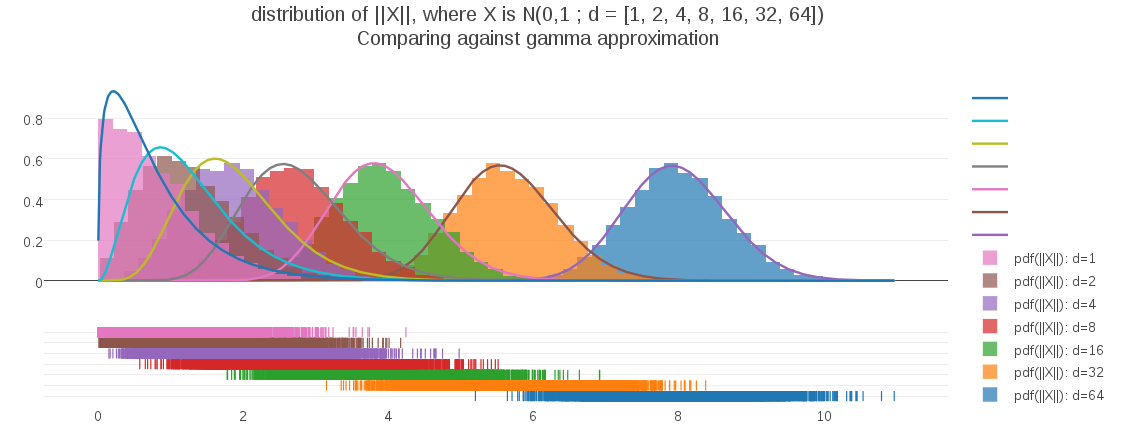As the plot shows, only for the 1-dimension case is the gamma approximation substiantially deviating. In fact, the fit appears to get better as dimensionality increases. To address the 1D case, I can easily test the fit of a half-gaussian as a possible model.

# Computing Derivatives of the Gamma Function

| Feedback

In this post I’ll describe a simple algorithm to compute the kth derivatives of the Gamma function.

I’ll start by showing a simple recursion relation for these derivatives, and then gives its derivation. The kth derivative of Gamma(x) can be computed as follows:The recursive formula for the Dk functions has an easy inductive proof: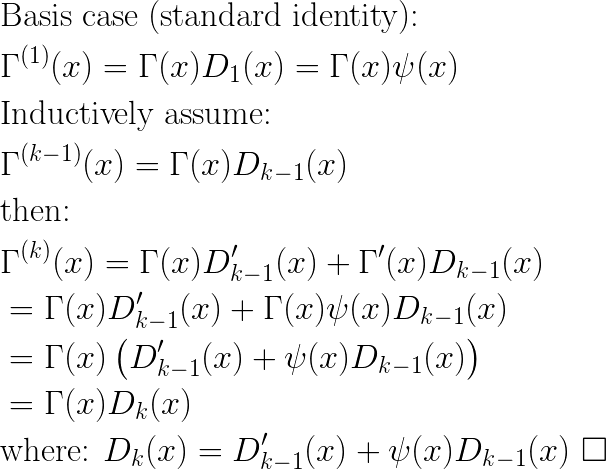Computing the next value Dk requires knowledge of Dk-1 but also derivative D’k-1. If we start expanding terms, we see the following:Continuing the process above it is not hard to see that we can continue expanding until we are left only with terms of D1(*)(x); that is, various derivatives of D1(x). Furthermore, each layer of substitutions adds an order to the derivatives, so that we will eventually be left with terms involving the derivatives of D1(x) up to the (k-1)th derivative. Note that these will all be successive orders of the polygamma function.

What we want, to do these computations systematically, is a formula for computing the nth derivative of a term Dk(x). Examining the first few such derivatives suggests a pattern: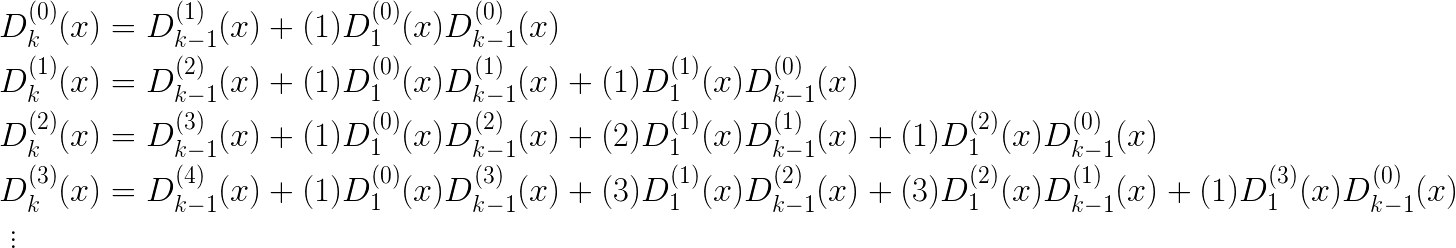Generalizing from the above, we see that the formula for the nth derivative is: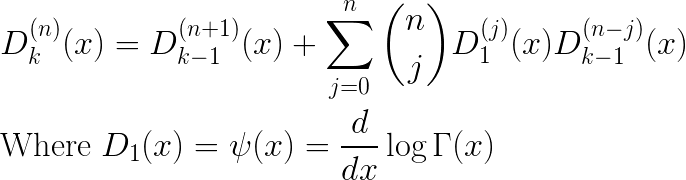We are now in a position to fill in the triangular table of values, culminating in the value of Dk(x):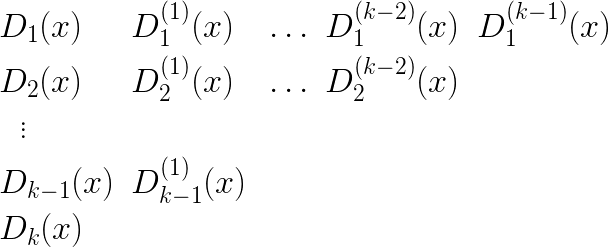As previously mentioned, the basis row of values D1(*)(x) are the polygamma functions where D1(n)(x) = polygamma(n)(x). The first two polygammas, order 0 and 1, are simply the digamma and trigamma functions, respectively, and are available with most numeric libraries. Computing the general polygamma is a project, and blog post, for another time, but the standard polynomial approximation for the digamma function can of course be differentiated… Happy Computing!

# Exploring the Effects of Dimensionality on a PDF of Distances

| Feedback

Every so often I’m reminded that the effects of changing dimensionality on objects and processes can be surprisingly counterintuitive. Recently I ran across a great example of this, while I working on a model for the distribution of distances in spaces of varying dimension.

Suppose that I draw some values from a classic one-dimensional Gaussian, with zero mean and unit variance, but that I am actually interested in their corresponding distances from center. Knowing that my Gaussian is centered on the origin, I can rephrase that as: the distribution of magnitudes of values drawn from that Gaussian. I can simulate this process by actually samping Gaussian values and taking their absolute value. When I do, I get the following result: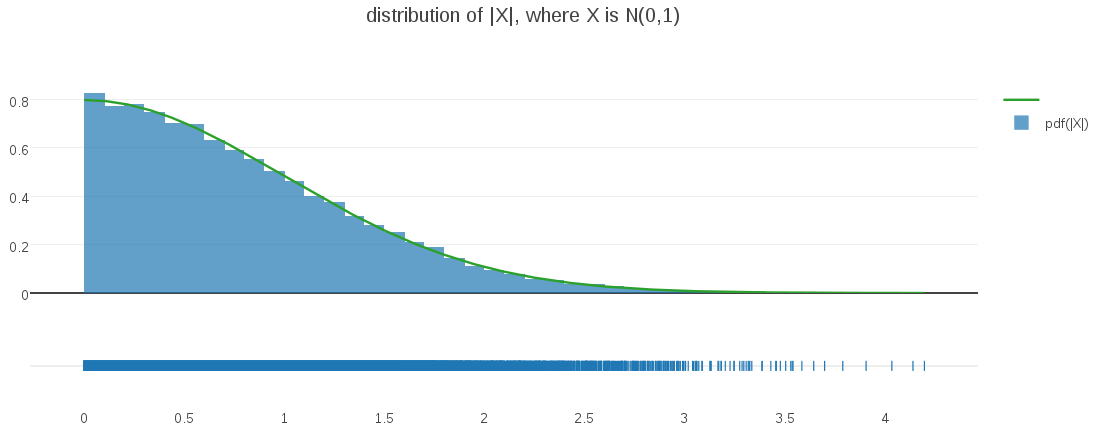It’s easy to see – and intuitive – that the resulting distribution is a half-Gaussian, as I confirmed by overlaying the histogrammed samples above with a half-Gaussian PDF (displayed in green).

I wanted to generalize this basic idea into some arbitrary dimensionality, (d), where I draw d-vectors from an d-dimensional Gaussian (again, centered on the origin with unit variances). When I take the magnitudes of these sampled d-vectors, what will the probability distribution of their magnitudes look like?

My intuitive assumption was that these magnitudes would also follow a half-Gaussian distribution. After all, every multivariate Gaussian is densest at its mean, just like the univariate case I examined above. In fact I was so confident in this assumption that I built my initial modeling around it. Great confusion ensued, when I saw how poorly my models were working on my higher-dimensional data!

Eventually it occurred to me to do the obvious thing and generate some visualizations from higher dimensional data. For example, here is the correponding plot generated from a bivariate Gaussian (d=2):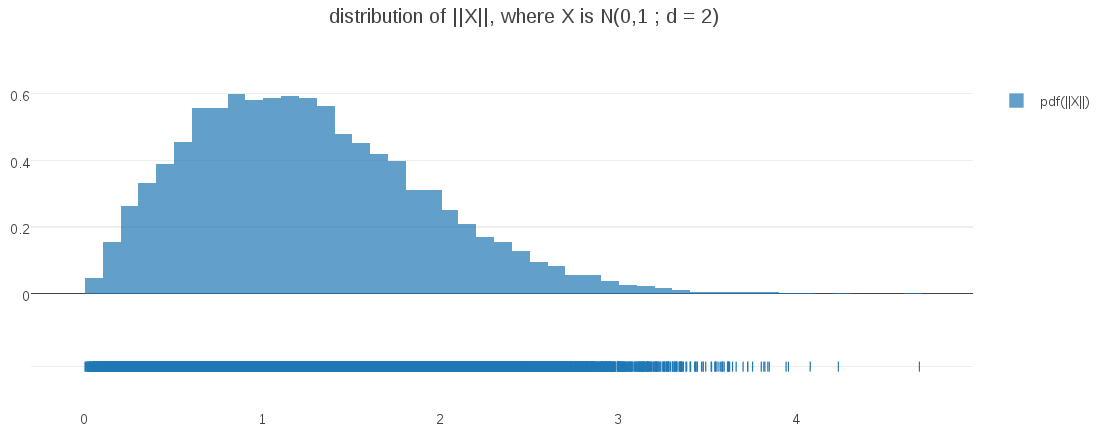Surprise – the distribution at d=2 is not even close to half-Gaussian!. My intuitions couldn’t have been more misleading!

Where did I go wrong?

I’ll start by observing what happens when I take a multi-dimensional PDF of vectors in (d) dimensions and project it down to a one-dimensional PDF of the corresponding vector magnitudes. To keep things simple, I will be assuming a multi-dimensional PDF fr(xd) that is (1) centered on the origin, and (2) is radially symmetric; the pdf value is the same for all points at a given distance from the origin. For example, any multivariate Gaussian with 0d mean and Id for a covariance matrix satisfies these two assumptions. With this in mind, you can see that the process of projecting from vectors in Rd to their distance from 0d (their magnitude) is equivalent to summing all densities fr(xd) along the surface of “d-sphere” radius (r) to obtain a pdf f(r) in distance space. With assumption (2) we can simplify that integration to just f(r)=Ad(r)f’(r), where f’(r) defines the value of fr(x) for all x with magnitude of (r), and Ad(r) is the surface area of a d-sphere with radius (r):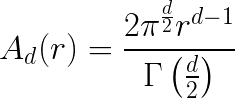The key observation is that this term is a polynomial function of radius (r), with degree (d-1). When d=1, it is simply a constant multiplier and so we get the half-Gaussian distribution we expect, but when d >= 2, the term is zero at r=0, and grows with radius. Hence we see the in the d=2 plot above that the density begins at zero, then grows with radius until the decreasing gaussian density gradually drives it back toward zero again.

The above ideas can be expressed compactly as follows: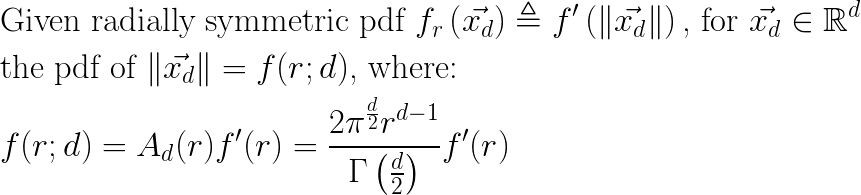In my experiments, I am using multivariate Gaussians of mean 0d and unit covariance matrix Id, and so the form for f(r;d) becomes:This form is in fact the generalized gamma distribution, with scale parameter a=21/2, shape parameter p=2, and free shape parameter (d) representing the dimensionality in this context.

I can verify that this PDF is correct by plotting it against randomly sampled data at differing dimensions: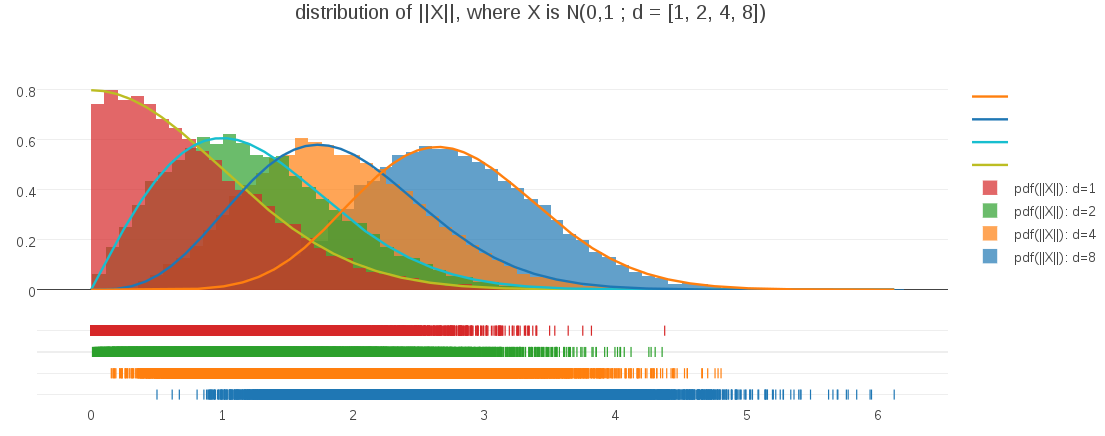This plot demonstrates both that the PDF expression is correct for varying dimensionalities and also illustrates how the shape of the PDF evolves as dimensionality changes. For me, it was a great example of challenging my intuitions and learning something completely unexpected about the interplay of distances and dimension.

# Measuring Decision Tree Split Quality with Test Statistic P-Values

| Feedback

When training a decision tree learning model (or an ensemble of such models) it is often nice to have a policy for deciding when a tree node can no longer be usefully split. There are a variety possibilities. For example, halting when node population size becomes smaller than some threshold is a simple and effective policy. Another approach is to halt when some measure of node purity fails to increase by some minimum threshold. The underlying concept is to have some measure of split quality, and to halt when no candidate split has sufficient quality.

In this post I am going to discuss some advantages to one of my favorite approaches to measuring split quality, which is to use a test statistic significance – aka “p-value” – of the null hypothesis that the left and right sub-populations are the same after the split. The idea is that if a split is of good quality, then it ought to have caused the sub-populations to the left and right of the split to be meaningfully different. That is to say: the null hypothesis (that they are the same) should be rejected with high confidence, i.e. a small p-value. What constitutes “small” is always context dependent, but popular p-values from applied statistics are 0.05, 0.01, 0.005, etc.

update – there is now an Apache Spark JIRA and a pull request for this feature

The remainder of this post is organized in the following sections:

##### Consistency

Test statistic p-values have some appealing properties as a split quality measure. The test statistic methodology has the advantage of working essentially the same way regardless of the particular test being used. We begin with two sample populations; in our case, these are the left and right sub-populations created by a candidate split. We want to assess whether these two populations have the same distribution (the null hypothesis) or different distributions. We measure some test statistic ‘S’ (Student’s t, Chi-Squared, etc). We then compute the probability that |S| >= the value we actually measured. This probability is commonly referred to as the p-value. The smaller the p-value, the less likely it is that our two populations are the same. In our case, we can interpret this as: a smaller p-value indicates a better quality split.

This consistent methodology has a couple advantages contributing to user experience (UX). If all measures of split quality work in the same way, then there is a lower cognitive load to move between measures once the user understands the common pattern of use. A second advantage is better “unit analysis.” Since all such quality measures take the form of p-values, there is no risk of a chosen quality measure getting mis-aligned with a corresponding quality threshold. They are all probabilities, on the interval [0,1], and “smaller threshold” always means “higher threshold of split quality.” By way of comparison, if an application is measuring entropy and then switches to using Gini impurity, these measures are in differing units and care has to be taken that the correct quality threshold is used in each case or the model training policy will be broken. Switching between differing statistical tests does not come with the same risk. A p-value quality threshold will have the same semantic regardless of which statistical test is being applied: probability that left and right sub-populations are the same, given the particular statistic being measured.

##### Awareness of Sample Size

Test statistics have another appealing property: many are “aware” of sample size in a way that captures the idea that the smaller the sample size, the larger the difference between populations should be to conclude a given significance. For one example, consider Welch’s t-test, the two-sample variation of the t distribution that applies well to comparing left and right sub populations of candidate decision tree splits: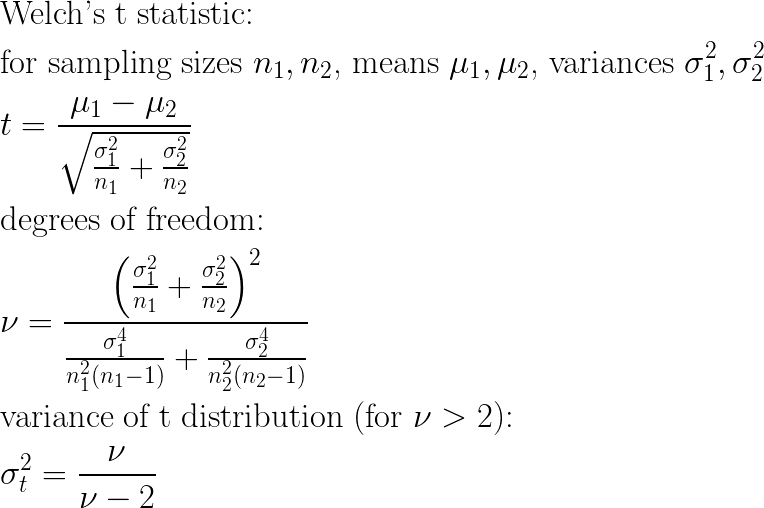Visualizing the effects of sample sizes n1 and n2 on these equations directly is a bit tricky, but assuming equal sample sizes and variances allows the equations to be simplified quite a bit, so that we can observe the effect of sample size: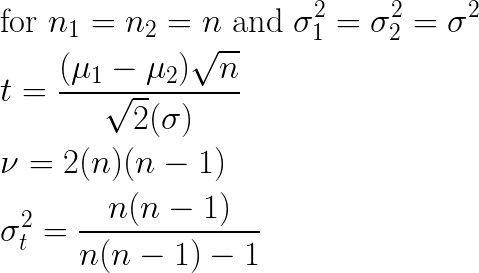These simplified equations show clearly that (all else remaining equal) as sample size grows smaller, the measured t-statistic correspondingly grows smaller (proportional to sqrt(n)), and furthermore the corresponding variance of the t distribution to be applied grows larger. For any given shift in left and right sub-populations, each of these trends yields a larger (i.e. weaker) p-value. This behavior is desirable for a split quality metric. The less data there is at a given candidate split, the less confidence there should be in split quality. Put another way: we would like to require a larger difference before a split is measured as being good quality when we have less data to work with, and that is exactly the behavior the t-test provides us.

##### Training Results

These propreties are pleasing, but it remains to show that test statistics can actually improve decision tree training in practice. In the following sections I will compare the effects of training with test statstics with other split quality policies based on entropy and gini index.

To conduct these experiments, I modified a local copy of Apache Spark with the Chi-Squared test statistic for comparing categorical distributions. The demo script, which I ran in spark-shell, can be viewed here.

I generated an example data set that represents a two-class learning problem, where labels may be 0 or 1. Each sample has 10 clean binary features, such that if the bit is 1, the probability of the label is 90% 1 and 10% 0. There are 5 noise features, also binary, which are completely random. There are 50 samples of each clean feature being on, for a total of 500 samples. There are also 500 samples where all clean features are 0 and the corresponding labels are 90% 0 and 10% 1. The total number of samples in the data set is 1000. The shape of the data is illustrated by the following table:

For the first run I use my customized chi-squared statistic as the split quality measure. I used a p-value threshold of 0.01 – that is, I would like my chi-squared test to conclude that the probability of left and right split populations are the same is <= 0.01, or that split will not be used. Note, this means I can expect that around 1% of the time, it will conclude a split was good, when it was just luck. This is a reasonable false-positive rate; random forests are by nature robust to noise, including noise in their own split decisions:

The first thing to observe is that the resulting decision tree used exactly the 10 clean features 0 through 9, and none of the five noise features. The tree splits off each of the clean features to obtain an optimally accurate leaf-node (one with 90% 1s and 10% 0s). A second observation is that the p-values shown in the demo output are extremely small (i.e. strong) values – around 1e-9 (one part in a billion) – for good-quality splits. We can also see “weak” p-values with magnitudes such as 0.7, 0.2, etc. These are poor quality splits on the noise features that it rejects and does not use in the tree, exactly as we hope to see.

Next, I will show a similar run with the standard available “entropy” quality measure, and a minimum gain threshold of 0.035, which is a value I had to determine by trial and error, as what kind of entropy gains one can expect to see, and where to cut them off, is somewhat unintuitive and likely to be very data dependent.

The first observation is that the resulting tree using entropy as a split quality measure is twice the size of the tree trained using the chi-squared policy. Worse, it is using the noise features – its quality measure is yielding many more false positives. The entropy-based model is less parsimonious and will also have performance problems since the model has included very noisy features.

Lastly, I ran a similar training using the “gini” impurity measure, and a 0.015 quality threshold (again, hopefully optimal value that I had to run multiple experiments to identify). Its quality is better than the entropy-based measure, but this model is still substantially larger than the chi-squared model, and it still uses some noise features:

##### Conclusion

In this post I have discussed some advantages of using test statstics and p-values as split quality metrics for decision tree training:

• Consistency
• Awareness of sample size
• Higher quality model training

I believe they are a useful tool for improved training of decision tree models! Happy computing!

# Random Forest Clustering of Machine Package Configurations in Apache Spark

| Feedback

In this post I am going to describe some results I obtained for clustering machines by which RPM packages that were installed on them. The clustering technique I used was Random Forest Clustering.

##### The Data

The data I clustered consisted of 135 machines, each with a list of installed RPM packages. The number of unique package names among all 135 machines was 4397. Each machine was assigned a vector of Boolean values: a value of 1 indicates that the corresponding RPM was installed on that machine. This means that the clustering data occupied a space of nearly 4400 dimensions. I discuss the implications of this later in the post, and what it has to do with Random Forest Clustering in particular.

For ease of navigation and digestion, the remainder of this post is organized in sections:

##### Random Forests and Random Forest Clustering

Full explainations of Random Forests and Random Forest Clustering could easily occupy blog posts of their own, but I will attempt to summarize them briefly here. Random Forest learning models per se are well covered in the machine learning community, and available in most machine learning toolkits. With that in mind, I will focus on their application to Random Forest Clustering, as it is less commonly used.

A Random Forest is an ensemble learning model, consisting of some number of individual decision trees, each trained on a random subset of the training data, and which choose from a random subset of candidate features when learning each internal decision node.

Random Forest Clustering begins by training a Random Forest to distinguish between the data to be clustered, and a corresponding synthetic data set created by sampling from the marginal distributions of each feature. If the data has well defined clusters in the joint feature space (a common scenario), then the model can identify these clusters as standing out from the more homogeneous distribution of synthetic data. A simple example of what this looks like in 2 dimensional data is displayed in Figure 1, where the dark red dots are the data to be clustered, and the lighter pink dots represent synthetic data generated from the marginal distributions: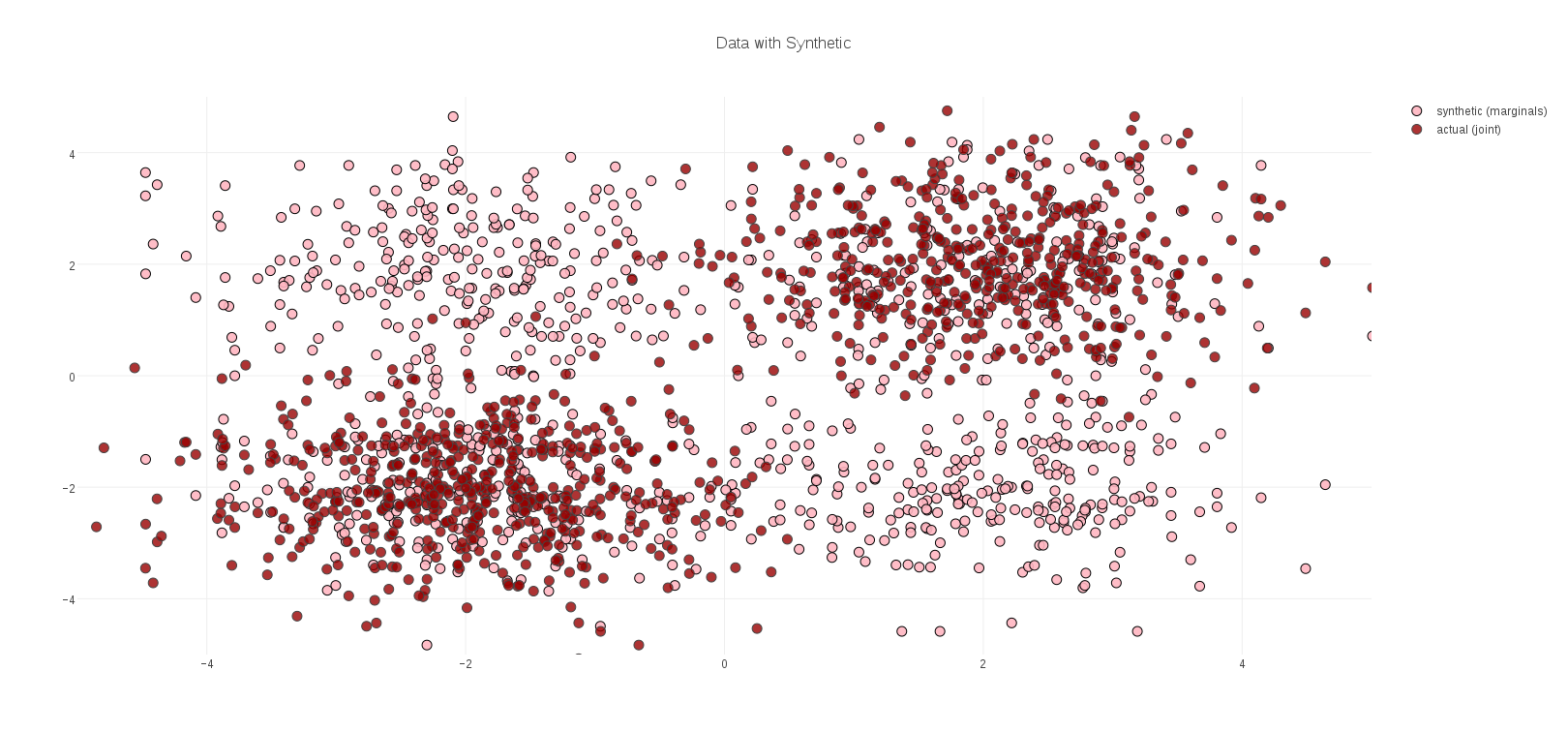Each interior decision node, in each tree of a Random Forest, typically divides the space of feature vectors in half: the half-space <= some threshold, and the half-space > that threshold. The result is that the model learned for our data can be visualized as rectilinear regions of space. In this simple example, these regions can be plotted directly over the data, and show that the Random Forest did indeed learn the location of the data clusters against the background of synthetic data: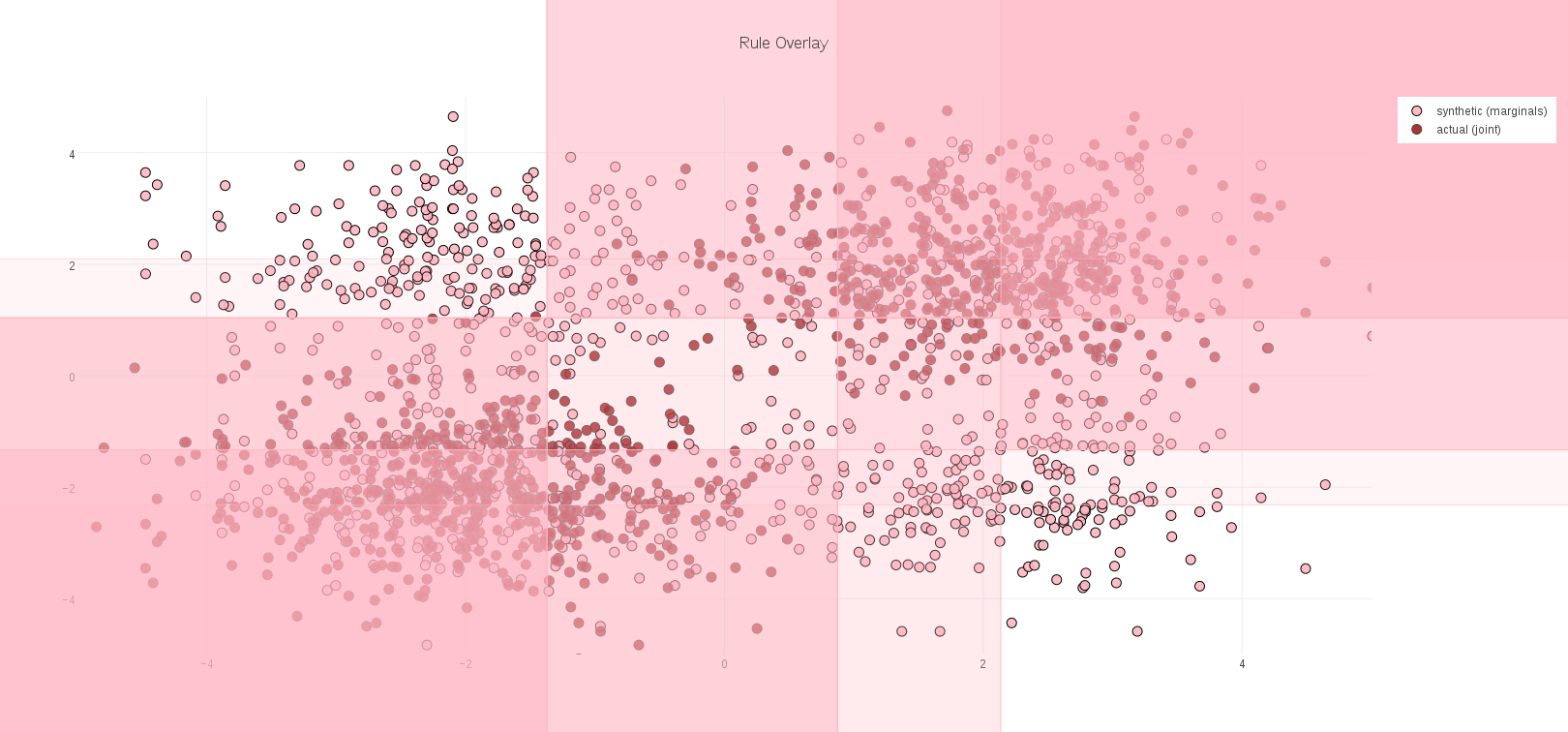Once this model has been trained, the actual data to be clustered are evaluated against this model. Each data element navigates the interior decision nodes and eventually arrives at a leaf-node of each tree in the Random Forest ensemble, as illustrated in the following schematic: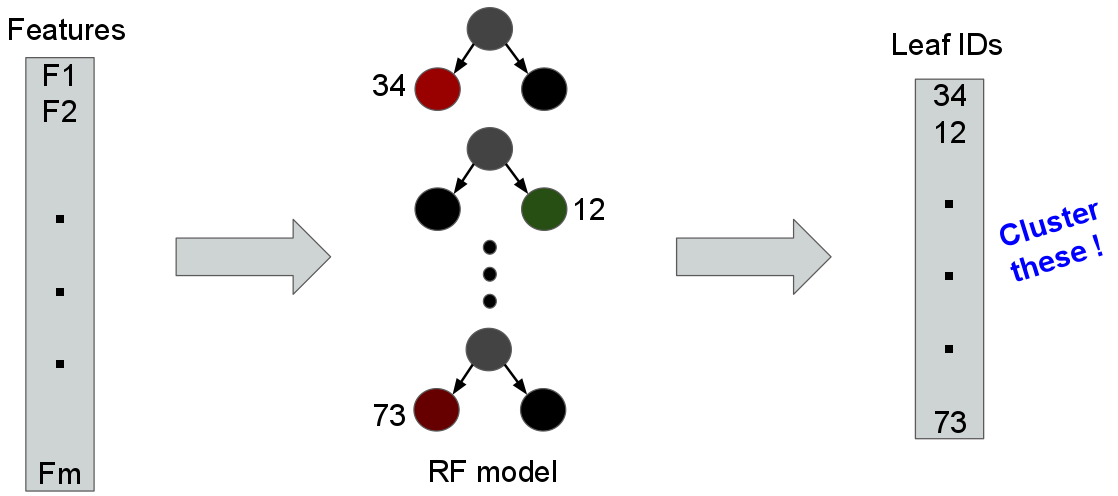A key insight of Random Forest Clustering is that if two objects (or, their feature vectors) are similar, then they are likely to arrive at the same leaf nodes more often than not. As the figure above suggests, it means we can cluster objects by their corresponding vectors of leaf nodes, instead of their raw feature vectors.

If we map the points in our toy example to leaf ids in this way, and then cluster the results, we obtain the following two clusters, which correspond well with the structure of the data: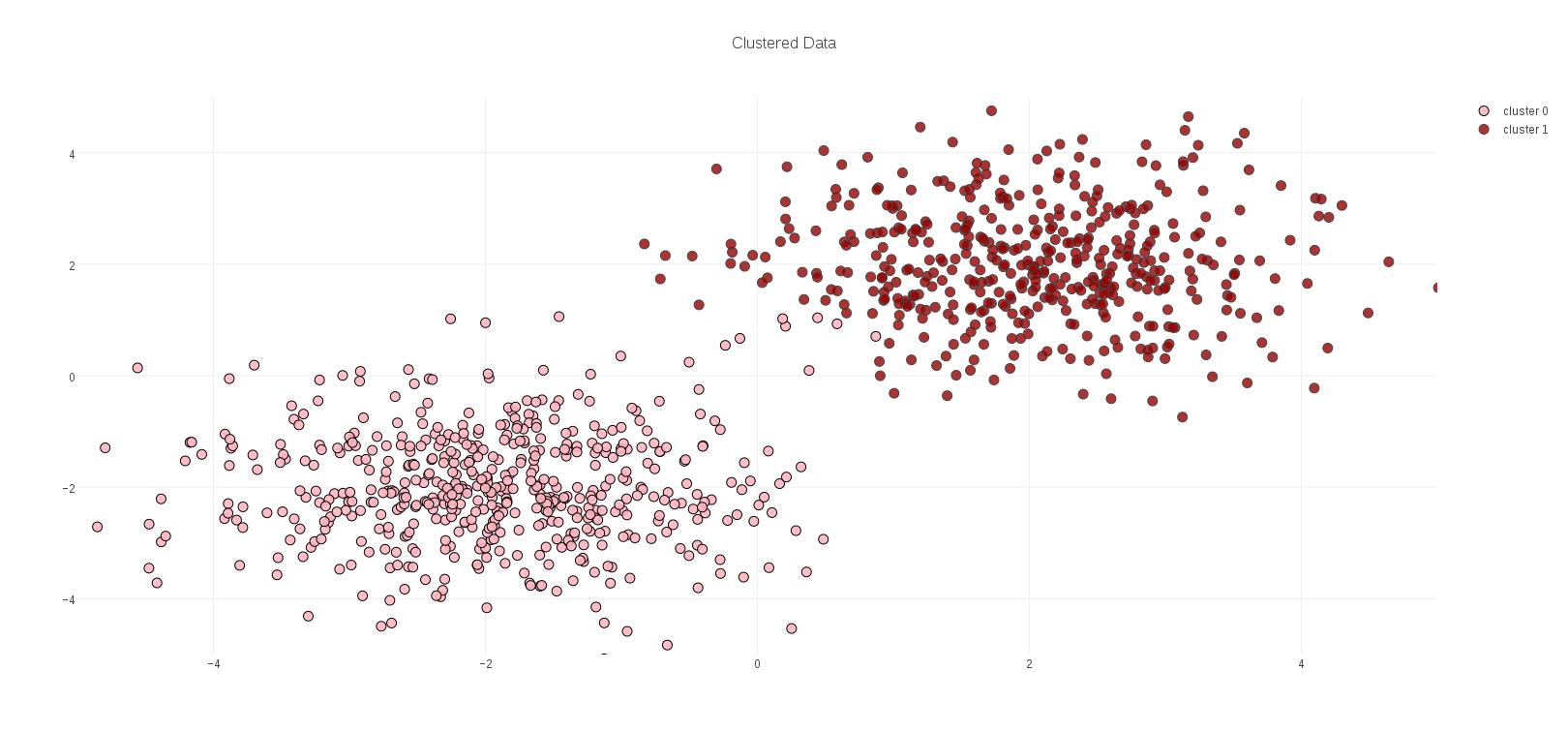A note on clustering leaf ids. A leaf id is just that – an identifier – and in that respect a vector of leaf ids has no algebra; it is not meaningful to take an average of such identifiers, any more than it would be meaningful to take the average of people’s names. Pragmatically, what this means is that the popular k-means clustering algorithm cannot be applied to this problem.

These vectors do, however, have distance: for any pair of vectors, add 1 for each corresponding pair of leaf ids that differ. If two data elements arrived at all the same leafs in the Random Forest model, all their leaf ids are the same, and their distance is zero (with respect to the model, they are the same). Therefore, we can apply k-medoids clustering.

##### The Pay-Off

What does this somewhat indirect method of clustering buy us? Why not just cluster objects by their raw feature vectors?

The problem is that in many real-world cases (unlike in our toy example above), feature vectors computed for objects have many dimensions – hundreds, thousands, perhaps millions – instead of the two dimensions in this example. Computing distances on such objects, necessary for clustering, is often expensive, and worse yet the quality of these distances is frequently poor due to the fact that most features in large spaces will be poorly correlated with any structure in the data. This problem is so common, and so important, it has a name: the Curse of Dimensionality.

Random Forest Clustering, which clusters on vectors of leaf-node ids from the trees in the model, side-steps the curse of dimensionality because the Random Forest training process, by learning where the data is against the background of the synthetic data, has already identified the features that are useful for identifying the structure of the data! If any particular feature was poorly correlated with that struture, it has already been ignored by the model. In other words, a Random Forest Clustering model is implicitly examining exactly those features that are most useful for clustering , thus providing a cure for the Curse of Dimensionality.

The machine package configurations whose clustering I describe for this post are a good example of high dimensional data that is vulnerable to the Curse of Dimensionality. The dimensionality of the feature space is nearly 4400, making distances between vectors potentially expensive to evaluate. Any individual feature contributes little to the distance, having to contend with over 4000 other features. Installed packages are also noisy. Many packages, such as kernels, are installed everywhere. Others may be installed but not used, making them potentially irrelevant to grouping machines. Furthermore, there are only 135 machines, and so there are far more features than data examples, making this an underdetermined data set.

All of these factors make the machine package configuration data a good test of the strenghts of Random Forest Clustering.

##### Package Configuration Clustering Code

The implementation of Random Forest Clustering I used for the results in this post is a library available from the silex project, a package of analytics libraries and utilities for Apache Spark.

In this section I will describe three code fragments that load the machine configuration data, perform a Random Forest clustering, and format some of the output. This is the code I ran to obtain the results described in the final section of this post.

The first fragment of code illustrates the logistics of loading the feature vectors from file train.txt that represent the installed-package configurations for each machine. A corresponding “parallel” file nodesclean.txt contains corresponding machine names for each vector. A third companion file rpms.txt contains names of each installed package. These are used to instantiate a specialized Scala function (InvertibleIndexFunction) between feature indexes and human-readable feature names (in this case, names of RPM packages). Finally, another specialized function (Extractor) for instantiating Spark feature vectors is created.

Note: Extractor and InvertibleIndexFunction are also component libraries of silex

The next section of code is where the work of Random Forest Clustering happens. A RandomForestCluster object is instantiated, and configured. Here, the configuration is for 7 clusters, 250 synthetic points (about twice as many synthetic points as true data), and a Random Forest of 20 trees. Training against the input data is a simple call to the run method.

The predictWithDistanceBy method is then applied to the data paired with machine names, to yield tuples of cluster-id, distance to cluster center, and the associated machine name. These tuples are split by distance into data with a cluster, and data considered to be “outliers” (i.e. elements far from any cluster center). Lastly, the histFeatures method is applied, to examine the Random Forest Model and identify any commonly-used features.

The final code fragment simply formats clusters and outliers into a tabular form, as displayed in the next section of this post. Note that there is neither Spark nor silex code here; standard Scala methods are sufficient to post-process the clustering data:

##### Package Configuration Clustering Results

The result of running the code in the previous section is seven clusters of machines. In the following files, the first column represents distance from the cluster center, and the second is the actual machine’s node name. A cluster distance of 0.0 indicates that the machine was indistinguishable from cluster center, as far as the Random Forest model was concerned. The larger the distance, the more different from the cluster’s center a machine was, in terms of its installed RPM packages.

Was the clustering meaningful? Examining the first two clusters below is promising; the machine names in these clusters are clearly similar, likely configured for some common task by the IT department. The first cluster of machines appears to be web servers and corresponding backend services. It would be unsurprising to find their RPM configurations were similar.

The second cluster is a series of executor machines of varying sizes, but presumably these would be configured similarly to one another.

The second pair of clusters (3 & 4) are small. All of their names are similar (and furthermore, similar to some machines in other clusters), and so an IT administrator might wonder why they ended up in oddball small clusters. Perhaps they have some spurious, non-standard packages installed that ought to be cleaned up. Identifying these kinds of structure in a clustering is one common clustering application.

Cluster 5 is a series of bugzilla web servers and corresponding back-end bugzilla data base services. Although they were clustered together, we see that the web servers have a larger distance from the center, indicating a somewhat different configuration.

Cluster 6 represents a group of performance-related machines. Not all of these machines occupy the same distance, even though most of their names are similar. These are also the same series of machines as in clusters 3 & 4. Does this indicate spurious package installations, or some other legitimate configuration difference? A question for the IT department…

Cluster 7 is by far the largest. It is primarily a combination of OpenStack machines and yet more perf machines. This clustering was relatively stable – it appeared across multiple independent clustering runs. Because of its stability I would suggest to an IT administrator that the performance and OpenStack machines are sharing some configuration similarities, and the performance machines in other clusters suggest that there might be yet more configuration anomalies. Perhaps these were OpenStack nodes that were re-purposed as performance machines? Yet another question for IT…

##### Outliers

This last grouping represents machines which were “far” from any of the previous cluster centers. They may be interpreted as “outliers” - machines that don’t fit any model category. Of these the node frodo is clearly somebody’s personal machine, likely with a customized or idiosyncratic package configuration. Unsurprising that it is farthest of all machines from any cluster, with distance 9.0. The jenkins machine is also somewhat unique among the nodes, and so perhaps not surprising that its registers as anomalous. The remaining machines match node series from other clusters. Their large distance is another indication of spurious configurations for IT to examine.

I will conclude with another useful feature of Random Forest Models, which is that you can interrogate them for information such as which features were used most frequently. Here is a histogram of model features (in this case, installed packages) that were used most frequently in the clustering model. This particular histogram i sinteresting, as no feature was used more than twice. The remaining features were all used exactly once. This is a bit unusual for a Random Forest model. Frequently some features are used commonly, with a longer tail. This histogram is rather “flat,” which may be a consequence of there being many more features (over 4000 installed packages) than there are data elements (135 machines). This makes the problem somewhat under-determined. To its credit, the model still achieves a meaningful clustering.

Lastly I’ll note that full histogram length was 186; in other words, of the nearly 4400 installed packages, the Random Forest model used only 186 of them – a tiny fraction! A nice illustration of Random Forest Clustering performing in the face of high dimensionality!

# Computing Simplex Vertex Locations From Pairwise Object Distances

| Feedback

Suppose I have a collection of (N) objects, and distances d(j,k) between each pair of objects (j) and (k); that is, my objects are members of a metric space. I have no knowledge about my objects, beyond these pair-wise distances. These objects could be construed as vertices in an (N-1) dimensional simplex. However, since I have no spatial information about my objects, I first need a way to assign spatial locations to each object, in vector space R(N-1), with only my object distances to work with.

In this post I will derive an algorithm for assigning vertex locations in R(N-1) for each of N objects, using only pairwise object distances.

I will assume that N >= 2, since at least two object are required to define a pairwise distance. The case N=2 is easy, as I can assign vertex 1 to the origin, and vertex 2 to the point d(1,2), to form a 1-simplex (i.e. a line segment) whose single edge is just the distance between the two objects. I will also assume that my N objects are distinct; that is, each pair has a non-zero distance.

Next consider an arbitrary N, and suppose I have already added vertices 1 through k. The next vertex (k+1) must obey the pairwise distance relations, as follows:Adding the new vertex (k+1) involves adding another dimension (k) to the simplex. I define this new kth coordinate x(k) to be zero for the existing k vertices, as annotated above; only the new vertex (k+1) will have a non-zero kth coordinate. Expanding the quadratic terms on the left yields the following form: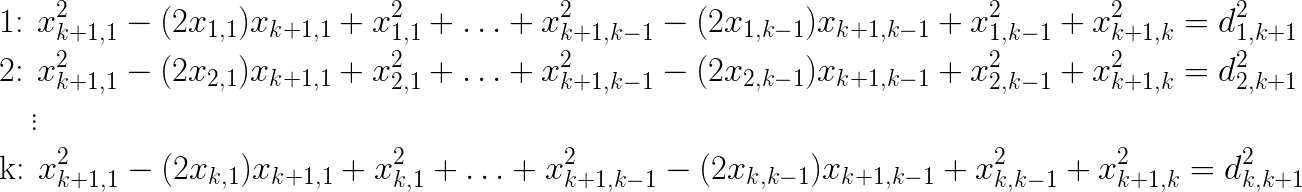The squared terms for the coordinates of the new vertex (k+1) are inconvenient, however I can get rid of them by subtracting pairs of equations above. For example, if I subtract equation 1 from the remaining k-1 equations (2 through k), these squared terms disappear, leaving me with the following system of k-1 equations, which we can see is linear in the 1st k-1 coordinates of the new vertex. Therefore, I know I’ll be able to solve for those coordinates. I can solve for the remaining kth coordinate by plugging it into the first distance equation: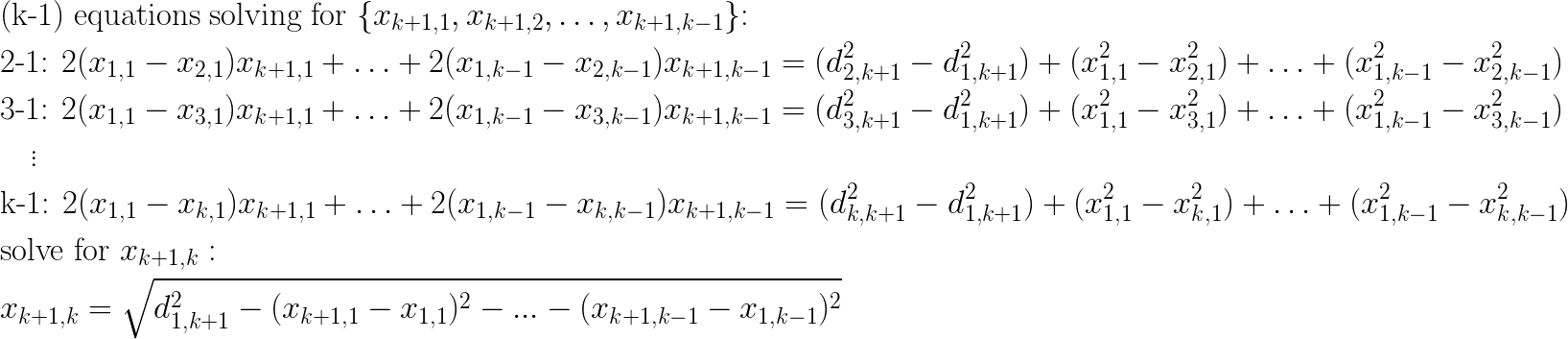To clarify matters, the equations above can be re-written as the following matrix equation, solveable by any linear systems library: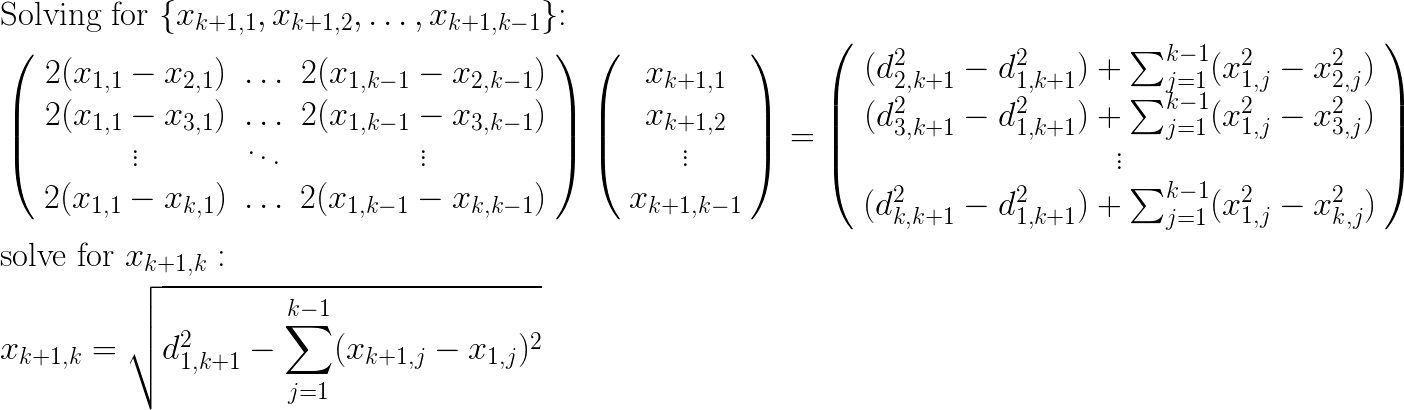This gives me a recusion relation for adding a new vertex (k+1), given that I have already added the first k vertices. The basis case of adding the first two vertices was already described above. And so I can iteratively add all my vertices one at a time by applying the recursion relation.

As a corollary, assume that I have constructed a simplex having k vertices, as shown above, and I would like to assign a spatial location to a new object, (y), given its k distances to each vertex. The corresponding distance relations are given by: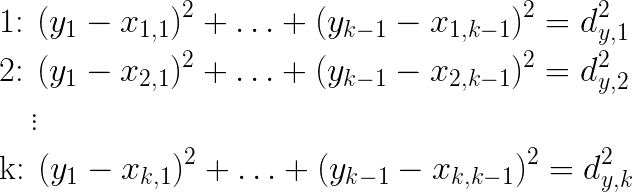I can apply a derivation very similar to the one above, to obtain the following linear equation for the (k-1) coordinates of (y):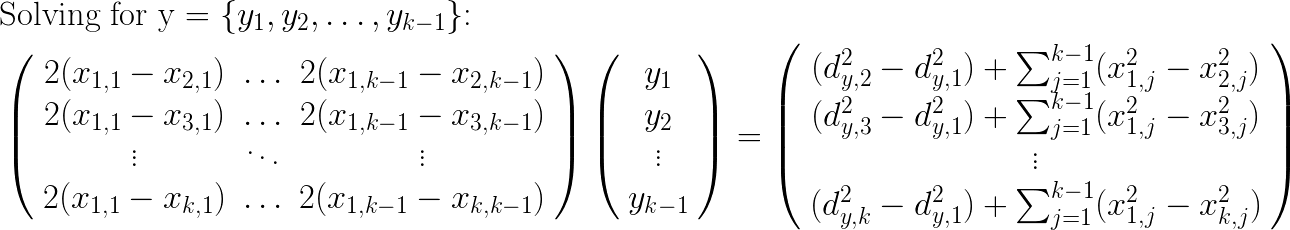# Efficient Multiplexing for Spark RDDs

| Feedback

In this post I’m going to propose a new abstract operation on Spark RDDsmultiplexing – that makes some categories of operations on RDDs both easier to program and in many cases much faster.

My main working example will be the operation of splitting a collection of data elements into N randomly-selected subsamples. This operation is quite common in machine learning, for the purpose of dividing data into a training and testing set, or the related task of creating folds for cross-validation).

Consider the current standard RDD method for accomplishing this task, randomSplit(). This method takes a collection of N weights, and returns N output RDDs, each of which contains a randomly-sampled subset of the input, proportional to the corresponding weight. The randomSplit() method generates the jth output by running a random number generator (RNG) for each input data element and accepting all elements which are in the corresponding jth (normalized) weight range. As a diagram, the process looks like this at each RDD partition: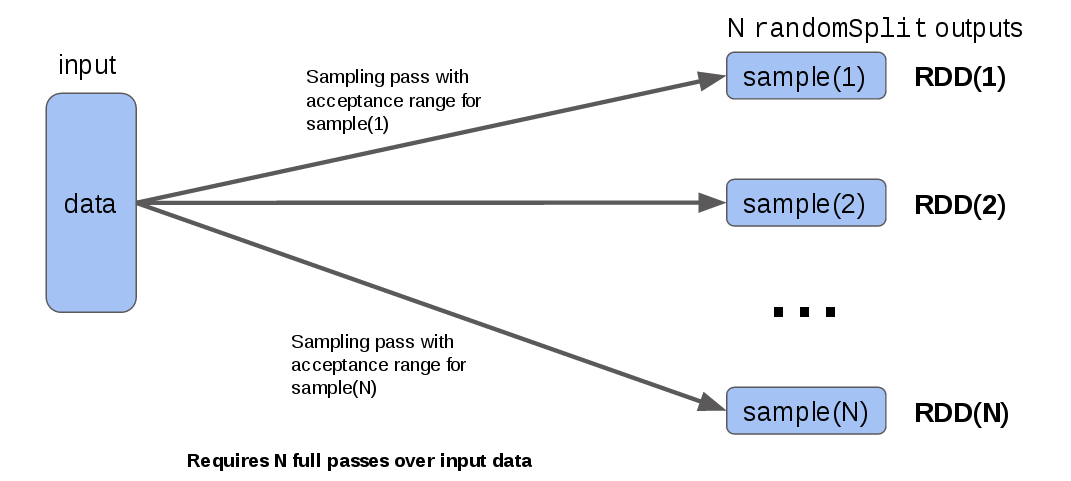The observation I want to draw attention to is that to produce the N output RDDs, it has to run a random sampling over every element in the input for each output. So if you are splitting into 10 outputs (e.g. for a 10-fold cross-validation), you are re-sampling your input 10 times, the only difference being that each output is created using a different acceptance range for the RNG output.

To see what this looks like in code, consider a simplified version of random splitting that just takes an integer n and always produces (n) equally-weighted outputs:

(Note that for this method to operate correctly, the RNG seed must be set to the same value each time, or the data will not be correctly partitioned)

While this approach to random splitting works fine, resampling the same data N times is somewhat wasteful. However, it is possible to re-organize the computation so that the input data is sampled only once. The idea is to run the RNG once per data element, and save the element into a randomly-chosen collection. To make this work in the RDD compute model, all N output collections reside in a single row of an intermediate RDD – a “manifold” RDD. Each output RDD then takes its data from the corresponding collection in the manifold RDD, as in this diagram: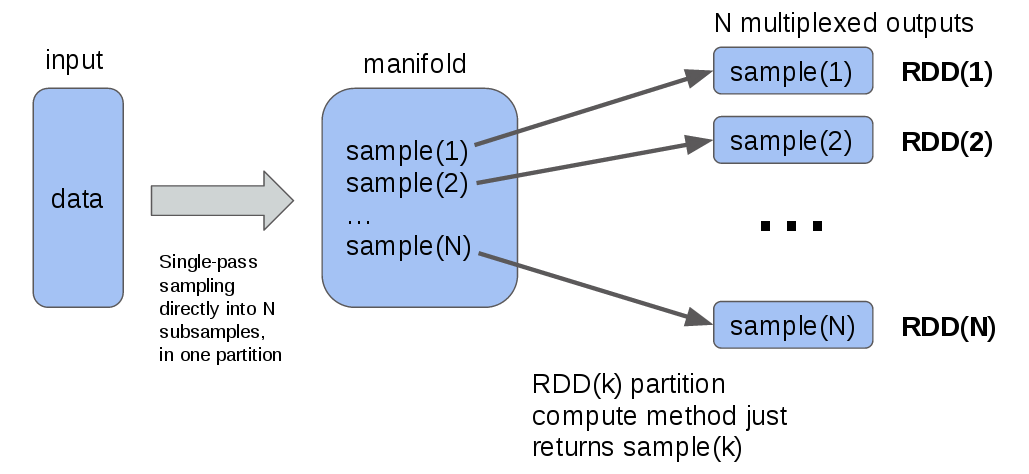If you abstract the diagram above into a generalized operation, you end up with methods that might like the following:

Here, the operation of sampling is generalized to any user-supplied function that maps RDD partition data into a sequence of objects that are computed in a single pass, and then multiplexed to the final user-visible outputs. Note that these functions take a StorageLevel argument that can be used to control the caching level of the internal “manifold” RDD. This typically defaults to MEMORY_ONLY, so that the computation can be saved and re-used for efficiency.

An efficient split-sampling method based on multiplexing, as described above, might be written using flatMuxPartitions as follows:

To test whether multiplexed RDDs actually improve compute efficiency, I collected run-time data at various split values of n (from 1 to 10), for both the non-multiplexing logic (equivalent to the standard randomSplit) and the multiplexed version: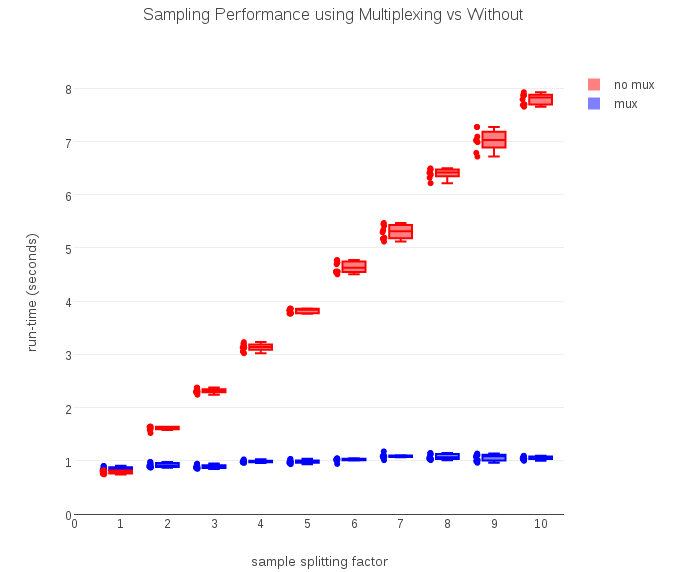As the timing data above show, the computation required to run a non-multiplexed version grows linearly with n, just as predicted. The multiplexed version, by computing the (n) outputs in a single pass, takes a nearly constant amount of time regardless of how many samples the input is split into.

There are other potential applications for multiplexed RDDs. Consider the following tuple-based versions of multiplexing:

Suppose you wanted to run an input-validation filter on some data, sending the data that pass validation into one RDD, and data that failed into a second RDD, paired with information about the error that occurred. Data validation is a potentially expensive operation. With multiplexing, you can easily write the filter to operate in a single efficient pass to obtain both the valid stream and the stream of error-data:

RDD multiplexing is currently a PR against the silex project. The code I used to run the timing experiments above is saved for posterity here.

Happy multiplexing!

# The ‘prepare’ operation considered harmful in Algebird aggregation

| Feedback

I want to make an argument that the Algebird Aggregator design, in particular its use of the prepare operation in a map-reduce context, has substantial inefficiencies, compared to an equivalent formulation that is more directly suited to taking advantage of Scala’s aggregate method on collections method.

Consider the definition of aggregation in the Aggregator class:

You can see that it is a standard map/reduce operation, where reduce is defined as a monoidal (or semigroup – more on this later) operation. Under the hood, it boils down to an invocation of Scala’s reduceLeft method. The key thing to notice is that the role of prepare is to map a collection of data elements into the required monoids, which are then aggregated using that monoid’s plus operation. In other words, prepare converts data elements into “singleton” monoids each representing a data element.

Now, if the monoid in question is simple, say some numeric type, this conversion is free, or nearly so. For example, the conversion of an integer into the “integer monoid” is a no-op. However, there are other kinds of “non-trivial” monoids, for which the conversion of a data element into its corresponding monoid may be costly. In this post, I will be using the monoid defined by Scala Set[Int], where the monoid plus operation is set union, and of course the zero element is the empty set.

Consider the process of defining an Algebird aggregator for the task of generating the set of unique elements in a data set. The corresponding prepare operation is: prepare(e: Int) = Set(e). A monoid trait that encodes this idea might look like the following. (the code I used in this post can be found here)

If we unpack the above code, as applied to intSetPrepared, we are instantiating a new Set object, containing a single value, for every single input data element.

But there is a potentially better model of aggregation, exemplified by the Scala aggregate method. This method does not use a prepare operation. It uses a zero value and a monoidal operator, which the Scala docs refer to as combop, but it also uses an “update” operation, that defines how to update the monoid object, directly, with a single element, referred to as seqop in Scala’s documentation. This idea can also be encoded as a flavor of monoid, enhanced with an update method:

This arrangement promises more efficiency when aggregating w.r.t. nontrivial monoids, by avoiding the construction of “singleton” monoids for each data element. The following demo confirms that for the Set-based monoid, it is over 10 times faster:

It is also possible to apply Scala’s aggregate to a monoid enhanced with prepare:

Although this turns out to be measurably faster than the literal map-reduce implementation, it is still not nearly as fast as the variation using update:

Readers familiar with Algebird may be wondering about my use of monoids above, when the Aggregator interface is actually based on semigroups. This is important, since building on Scala’s aggregate function requires a zero element that semigroups do not have. Although I believe it might be worth considering changing Aggregator to use monoids, another sensible option is to change the internal logic for the subclass AggregatorMonoid, which does require a monoid, or possibly just define a new AggregatorMonoidUpdated subclass.

A final note on compatability: note that any monoid enhanced with prepare can be converted into an equivalent monoid enhanced with update, as demonstrated by this factory function: